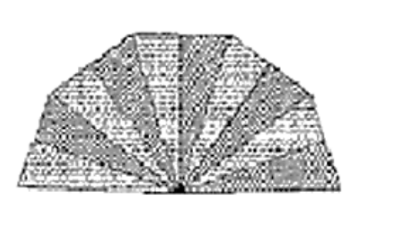# A hand fan is made by sticking 10 equal size triangular strips of two different types of paper as shown in the figure.

Question:

A hand fan is made by sticking 10 equal size triangular strips of two different types of paper as shown in the figure. The dimensions of equal strips are 25 cm, 25 cm and 14 cm. Find the area of each type of paper needed to make the hand fan.Solution:

Given that,

The sides of AOB

AO = 25 cm

OB = 25 cm

BA = 14 cm

Area of each strip = Area of triangle AOB

Now, for the area of triangle AOB

Perimeter = AO + OB + BA

2s = 25 cm +25 cm + 14 cm

s = 32 cm

By using Heron's Formula,

Area of the triangle $A O B=\sqrt{s \times(s-a) \times(s-b) \times(s-c)}$

$=\sqrt{32 \times(7) \times(4) \times(18)}$

$=168 \mathrm{~cm}^{2}$

Also, area of each type of paper needed to make a fan = 5 × Area of triangle AOB

Area of each type of paper needed to make a fan $=5 \times 168 \mathrm{~cm}^{2}$

Area of each type of paper needed to make a fan $=840 \mathrm{~cm}^{2}$